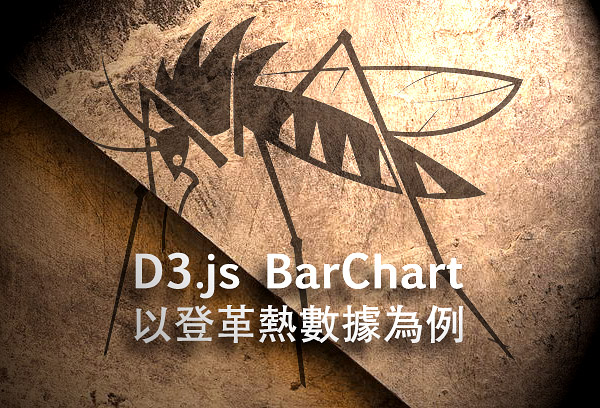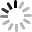# SVG D3.js - 直條圖 ( 以登革熱數據為例 )``````d3.csv("tainan-dengue.csv", function(data) {
var day = d3.nest()
.key(function(d){
return d.確診日;
})
.entries(data);

for(var i=0; i<day.length; i++){
if(i<9){
day[i]._id = '0'+(i+1).toString();
}else{
day[i]._id = (i+1).toString();
}
}

var dayById = d3.nest()
.key(function(d){
return d._id;
}).sortKeys(d3.descending)
.entries(day);

for(var j=0; j<dayById.length; j++){
dayById[j]._id = j+1;
}

console.log(dayById);
````````````var s = d3.select('body').append('svg')
.attr({
'width':800,
'height':day.length*15+20
}).style({
'border':'1px solid #000'
});
var rect = s.append('g')
.attr({
'id':'rect'
});
var num = s.append('g')
.attr({
'id':'num'
});
var date = s.append('g')
.attr({
'id':'date'
});
``````

``````rect.selectAll('rect')
.data(dayById)
.enter()
.append('rect')
.attr({
'width':function(d){
return d.values.values.length;
},
'height':10,
'fill':function(d){
if(d.values.values.length>300){
return '#c00';
}else if(d.values.values.length>200&&d.values.values.length<=300){
return '#f90';
}else if(d.values.values.length>100&&d.values.values.length<=200){
return '#aa0';
}else if(d.values.values.length>50&&d.values.values.length<=100){
return '#ac0';
}else{
return '#6c0';
}
},
'x':100,
'y':function(d){
return d._id*15;
}
});
``````

``````num.selectAll('text')
.data(dayById)
.enter()
.append('text')
.attr({
'fill':'#000',
'x':function(d){
return d.values.values.length+105;
},
'y':function(d){
return d._id * 15 + 10;
}
}).text(function(d){
return d.values.values.length;
}).style({
'font-size':'12px'
});
``````

``````date.selectAll('text')
.data(dayById)
.enter()
.append('text')
.attr({
'fill':'#000',
'text-anchor': 'end',
'x':90,
'y':function(d){
console.log(d);
return d._id * 15 + 10;
}
}).text(function(d){
return d.values.values.確診日;
}).style({
'font-size':'12px'
});
````````````d3.csv("tainan-dengue.csv", function(data) {

var area = d3.nest()
.key(function(d){
return d.區別;
})
.entries(data);

console.log(area);

d3.select('#button').selectAll('button')
.data(area)
.enter()
.append('button')
.text(function(d){
return d.key + ' ( ' +d.values.length+ ' )';
}).style({
'margin':'2px',
}).on('click',function(d){
d3.selectAll('button').style({
'background':'#ddd',
'color':'#000'
});
this.style.background = '#666';
this.style.color = '#fff';
});

function draw(drawData){

d3.selectAll('svg').remove();

//剛剛做的事情

}
});
``````